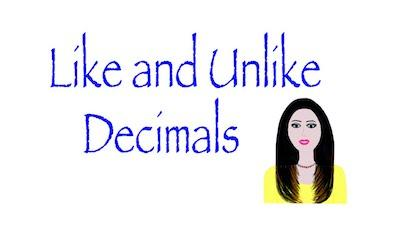# How To Convert Unlike Decimals To Like Decimals

When you start learning decimals, it is normal that your teacher tells you about the difference between unlike decimals and like decimals. While both definitions are quite easy to understand, some students find some difficulties in putting them into practice especially when they are required to convert unlike decimals to like decimals.

Discover everything you need to know about rounding numbers.

Before we show you how to convert unlike decimals to like decimals, it is important to know what like decimals and unlike decimals are in the first place.

## Like Decimals

Like decimals are simply the decimals that have the same number of decimal places. So, you can say that decimals that have the same number of decimal places are known as like decimals.

Here are some examples:

Decimals such as 56.8, 7.1, 36.1, 36.9, 0.8, 241.2 all are Like Decimals because:

• 56.8 has one decimal place i.e. digit 8
• 7.1 has one decimal place i.e. digit 1
• 36.1 has one decimal place i.e. digit 1
• 36.9 has one decimal place i.e. digit 9
• 0.8 has one decimal place i.e. digit 8
• 241.2 has one decimal place i.e. digit 2

As you can see, all these decimals only have one decimal place. So, they are known as like decimals. And this is also the case of the decimals 54.32, 0.82, 4.78, 919.32 and 14.93 which all have two decimal places and they are also called like decimals.

Discover how to round decimals to the nearest whole number.

## Unlike Decimals

Unlike decimals are the decimals that have a different number of decimal places. So, we can then say that decimals that have a different number of decimal places are known as unlike decimals.

Here are some examples:

Decimals such as 56.8, 7.12, 36.1423, 0.821 all are unlike decimals because:

• 56.8 has one decimal place i.e. digit 8
• 7.12 has two decimal places i.e. digit 1 & 2
• 36.1423 has four decimal places i.e. digit 1, 4, 2 and 3
• 0.821 has three decimal places i.e. digit 8, 2 and 1

Since all the previous decimals have different decimal places, we can then say that we are looking at unlike decimals.

Discover the rules to round decimal numbers.

## How To Convert Unlike Decimals To Like Decimals

Now that you understand the difference between like and unlike decimals, it is time to see how you can easily convert unlike decimals to like decimals. To do so, you just need to follow the next steps:

Step #1: Find the decimal fraction with the greatest numbers of decimal places.

Step #2: Now, make other given decimal fractions/ decimal numbers with the same number of decimal places by adding zeroes to the right of last digit of decimal part so that each decimal fraction has decimal places equal to the greatest number of decimal places.

Notice that you can do this because adding zeros on the right of the digit of the decimal part doesn’t change the value of the decimal number.

Here’s an example: Let’s say that you have the decimals 7.1 and 18.34 and you want to convert unlike decimals to like decimals.

As you can see, while the first decimal only has 1 decimal place, the second one has 2 decimal places. So, what you need to do is to add one zero to the extreme right of the first decimal 7.1. This will make this decimal look like this: 7.10.

So, after the conversion, you get:

7.10 that has two decimal places i.e. digit 1 and 0

Also, 7.1 = 7.10 i.e. Value of the decimal is not affected.

So, now you have 7.10 and 18.34 which are now like decimals because they have the same number of decimal places.